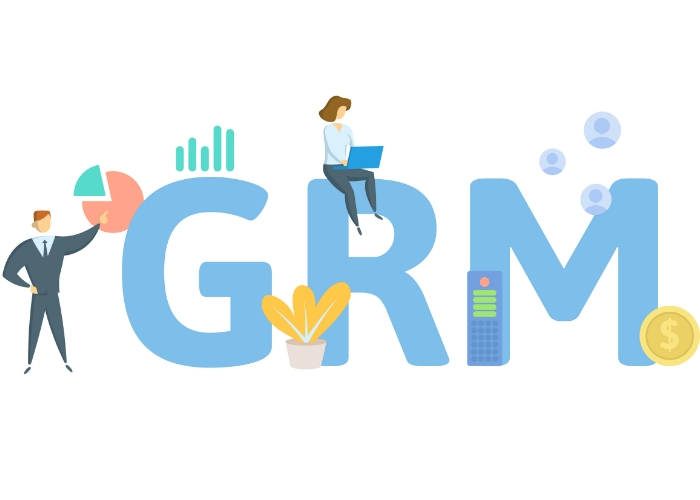# Gross Rent Multiplier (GRM): Real Estate Investor’s Guide#### IN THIS GUIDE

The gross rent multiplier (GRM) is a popular metric used by real estate investors to quickly estimate the value of an income-producing property. GRMs can vary widely depending on the location of the property, type of property, and other factors. To calculate the GRM, simply divide the purchase price of a property by the annual gross rents collected. The lower the GRM, the better the deal is for the investor.

## What Is GRM in Real Estate?

GRM is a simple way to estimate the value of an income-producing property, because it measures the number of years required for a property to produce income equal to its purchase price. It is calculated by dividing the sale price of a property by the annual gross rental income. GRMs can vary widely depending on the location of the property, type of property, and other factors.

In general, properties with lower GRMs are considered to be better values, because they generate more income relative to their purchase price. That said, the average GRM is between 4 and 7. The GRM is just one factor to consider when evaluating an investment property; other factors such as location and condition of the property also play a role in determining its value.

## How to Calculate GRM in Real Estate: GRM Formula

To calculate the GRM, simply divide the purchase price of a property by the annual gross rents collected.

GRM = Purchase Price / Annual Gross Rents

Take, for example, a rental property that costs \$200,000 and generates \$20,000 in gross annual rents. Based on these numbers, the GRM for the property is 10.

### What is GRM vs GIM?

The gross income multiplier (GIM) is very similar to the GRM, except that it takes into account all of the income generated by a property, not just the rent. This includes things like laundry income, parking income, and any other miscellaneous revenue. For that reason, the GIM is a more accurate measure of a property’s true earning potential.

Calculate the GIM for a piece of commercial real estate by dividing the purchase price by the total annual income it will generate.

GIM = Purchase Price / Total Annual Income

Based on the same example above, a rental property that costs \$200,000 and generates \$20,000 in gross annual rents plus \$5,000 in other miscellaneous income would have a GIM of 8.

## What is a Good Gross Rent Multiplier?

Income producing properties with a low gross rent multiplier present a better investment opportunity than those with higher GRMs because it takes longer for a high-GRM investment to pay for itself through rental income.

That said, what constitutes a high GRM varies depending on the location and type of property. In general, though, a GRM over 10 is considered high, while a GRM of 4 is considered low.

## How to Use GRM in Real Estate Investing

GRM can be a helpful tool for real estate investing because it gives you a quick estimate of what a property is worth. However, it’s important to remember that the GRM is just one metric and should not be the only factor considered.

When analyzing a potential investment property, look at other factors such as the property’s cash flow, cap rate, and return on investment (ROI). These factors will give you a more complete picture of whether or not a particular property is a good investment.

## Cap Rate vs. GRM

Capitalization rate (cap rate) is another metric that is often used by real estate investors. The cap rate is a measure of the potential return on investment (ROI) of a property, and essentially is the inverse of GRM. Whereas a lower GRM is ideal for investors, a higher cap rate signals a better investment. Unlike the GRM, the cap rate of an investment property is expressed as a percentage of the property’s value or purchase price.

The main difference between GRM and cap rate is that GRM only takes into account the gross income of a property, while the cap rate takes into account the net operating income (NOI). Because of this difference, the cap rate is generally a more accurate measure of a property’s profitability and investment potential.

To calculate the cap rate, simply divide the NOI by the purchase price of the property.

Cap Rate = Net Operating Income / Purchase Price

For example, if you buy a rental property for \$200,000 and it generates \$20,000 in annual NOI, then the cap rate for that property is 10%.